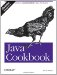# Recipe 7.8 Sorting a Collection

#### Problem

You put your data into a collection in random order or used a Properties object that doesn't preserve the order, and now you want it sorted.

#### Solution

Use the static method Arrays.sort( ) or Collections.sort( ), optionally providing a Comparator.

#### Discussion

If your data is in an array, you can sort it using the static sort( ) method of the Arrays utility class. If it is in a Collection, you can use the static sort( ) method of the Collections class. Here is a set of strings being sorted, first in an Array and then in a Vector:

`public class SortArray {     public static void main(String[] unused) {         String[] strings = {             "painful",              "mainly",             "gaining",             "raindrops"         };         Arrays.sort(strings);         for (int i=0; i<strings.length; i++)             System.out.println(strings[i]);     } } public class SortCollection {     public static void main(String[] unused) {         List l ist = new ArrayList( );         list.add("painful");         list.add("mainly");         list.add("gaining");         list.add("raindrops");                  Collections.sort(list);         for (int i=0; i<list.size( ); i++)             System.out.println(list.elementAt(i));     } }`

What if the default sort order isn't what you want? Well, you can create an object that implements the Comparator interface and pass that as the second argument to sort. Fortunately, for the most common ordering next to the default, you don't have to: a public constant String.CASE_INSENSITIVE_ORDER can be passed as this second argument. The String class defines it as "a Comparator that orders String objects as by compareToIgnoreCase." But if you need something fancier, you probably need to write a Comparator. Suppose that, for some strange reason, you need to sort strings using all but the first character of the string. One way to do this would be to write this Comparator:

`public class SubstringComparator  implements Comparator {     public int compare(Object o1, Object o2) {         String s1 = o1.toString( ).substring(1);         String s2 = o2.toString( ).substring(1);         return s1.compareTo(s2);         // or, more concisely:         // return o1.toString( ).substring(1).equals(o2toString( )..substring(1));     } }`

Using it is just a matter of passing it as the Comparator argument to the correct form of sort( ), as shown here:

`import java.util.*; public class SubstrCompDemo {     public static void main(String[] unused) {         String[] strings = {             "painful",              "mainly",             "gaining",             "raindrops"         };         Arrays.sort(strings);         dump(strings, "Using Default Sort");         Arrays.sort(strings, new SubstringComparator( ));         dump(strings, "Using SubstringComparator");     }     static void dump(String[] args, String title) {         System.out.println(title);         for (int i=0; i<args.length; i++)             System.out.println(args[i]);     } }`

Here is the output of running it:

`\$ java  SubstrCompDemo Using Default Sort gaining mainly painful raindrops Using SubstringComparator raindrops painful gaining mainly`

And this is all as it should be.

On the other hand, you may be writing a class and want to build in the comparison functionality so that you don't always have to remember to pass the Comparator with it. In this case, you can directly implement the java.lang.Comparable interface. The String class; the wrapper classes Byte, Character, Double, Float, Long, Short, and Integer, BigInteger and BigDecimal from java.math; File from java.io; java.util.Date; and java.text.CollationKey all implement this interface, so arrays or Collections of these types can be sorted without providing a Comparator. Classes that implement Comparable are said to have a " natural" ordering. The documentation strongly recommends that a class's natural ordering be consistent with its equals( ) method, and it is consistent with equals( ) if and only if e1.compareTo((Object)e2)==0 has the same Boolean value as e1.equals((Object)e2) for every instance e1 and e2 of the given class. This means that if you implement Comparable, you should also implement equals( ), and the logic of equals( ) should be consistent with the logic of the compareTo( ) method. Here, for example, is part of the appointment class Appt from a hypothetical scheduling program:

`public class Appt implements Comparable {     // much code and variables omitted - see online version     //-----------------------------------------------------------------     //    METHODS - COMPARISON     //-----------------------------------------------------------------     /** compareTo method, from Comparable interface.      * Compare this Appointment against another, for purposes of sorting.      * <P>Only date and time participate, not repetition!      * Consistent with equals( ).      * @return -1 if this<a2, +1 if this>a2, else 0.      */      public int compareTo(Object o2) {         Appt a2 = (Appt) o2;         if (year < a2.year)             return -1;         if (year > a2.year)             return +1;         if (month < a2.month)             return -1;         if (month > a2.month)             return +1;         if (day < a2.day)             return -1;         if (day > a2.day)             return +1;         if (hour < a2.hour)             return -1;         if (hour > a2.hour)             return +1;         if (minute < a2.minute)             return -1;         if (minute > a2.minute)             return +1;         return target.compareTo(a2.target);     }     /** Compare this appointment against another, for equality.      * Consistent with compareTo( ). For this reason, only      * date & time participate, not repetition.      * @returns true if the objects are equal, false if not.      */     public boolean equals(Object o2) {         Appt a2 = (Appt) o2;         if (year != a2.year ||             month != a2.month ||             day != a2.day ||             hour != a2.hour ||             minute != a2.minute)             return false;         return target.equals(a2.target);     }`

If you're still confused between Comparable and Comparator, you're probably not alone. This table summarizes the two "comparison" interfaces:

Interface name

Description

Method(s)

`java.lang.Comparable`

Provides a natural order to objects. Used in the class whose objects are being sorted.

`int compareTo(Object o); boolean equals(Object c2)`

`java.util.Comparator`

Provides total control over sorting objects of another class. Standalone; pass to sort( ) method or Collection constructor. Implements Strategy Design Pattern.

`int compare(Object o1, Object o2);`Java Cookbook, Second Edition
ISBN: 0596007019
EAN: 2147483647
Year: 2003
Pages: 409
Authors: Ian F Darwin

Similar book on Amazon